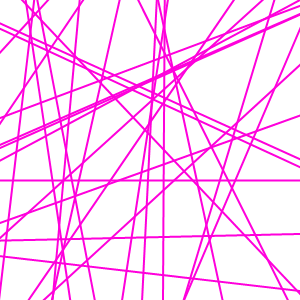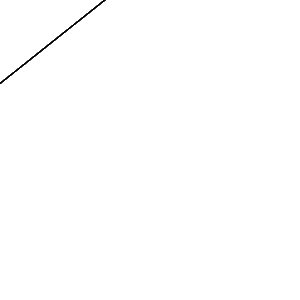Recommended Posts

Hey y'all, I've seen a lot of work starting with drawing random lines on the screen, and this time I'm doing some of my own that way. But making enough lines tends to be very long. So I was wondering whether someone could make a plugin that would have:

1 slider to adjust the amount of lines

1 slider to choose the thickness of the lines

1 slider for antialiasing.

Thanks,

~Blooper

Share on other sites
Have you tried drawing a line and using Ed Harvey's Fragment?

Kinda throws out the "random" part of it, eh...?Share on other sites

Full screen barcode plugin? Just type in something, and it'll generate seemingly random lines.

Share on other sites

Add noise and motion blur is just about as slow as making lines with the line tool. An example:The lines are all the same thickness, but they're not going to the same place.

Share on other sites

That's a tricky thing to code...

Paint.net works per threads. Meaning the image is cut in smaller sub-image and the program runs separatly on each of them.

This is why I create first the table of coords X,Y one time for the whole image and then use the table so lines definition are unique...

(perhaps not clear to everyone...NM)

This is the code for CodeLab:

int Amount1=50;    //[1,1000]Quantity
int Amount2=1;     //[0,1]Clamp to borders 0:no,1:yes
int Amount3=3;     //[1,200]Line width

bool init = false;
int sAmount1=-1;
int sAmount2=-1;
int sAmount3=-1;
int randgen = 0;

public static Random Rand = new Random();

void Render(Surface dst, Surface src, Rectangle rect)
{
PdnRegion selectionRegion = EnvironmentParameters.GetSelection(src.Bounds);
Rectangle selection = this.EnvironmentParameters.GetSelection(src.Bounds).GetBoundsInt();

int MaxiX = (selection.Right - selection.Left);
int MaxiY = (selection.Bottom - selection.Top);

int MaxiP = Amount1;
if ((!init) || (sAmount1 != Amount1) || (sAmount2 != Amount2) || (sAmount3 != Amount3))
{
// Set random generator
Random randinit = new Random();
if (randgen == 0) randgen = randinit.Next(1 + Math.Abs(unchecked(System.Threading.Thread.CurrentThread.GetHashCode() ^ unchecked((int)DateTime.Now.Ticks))));
Rand = new Random(randgen);
init = false;
sAmount1 = Amount1;
sAmount2 = Amount2;
sAmount3 = Amount3;
}
double Period  = MaxiX/Amount1;
double Scale  = (Amount2 / 100.0) * (double)MaxiY / (double)MaxiX;
double Angle = 0;
double s = 0;
long CenterX = (long)(((selection.Right - selection.Left) / 2)+selection.Left);
long CenterY = (long)(((selection.Bottom - selection.Top) / 2)+selection.Top);

ColorBgra PrimColor = (ColorBgra)EnvironmentParameters.PrimaryColor;
int BrushWidth = Amount3;

SolidBrush Brush1 = new SolidBrush(Color.FromArgb(PrimColor.A, PrimColor.R, PrimColor.G, PrimColor.);
Pen Pen1 = new Pen(Brush1, BrushWidth);

// Calculate the X,Y coords of the curve
int[] sx1 = new int[1 + MaxiP];
int[] sy1 = new int[1 + MaxiP];
int[] sx2 = new int[1 + MaxiP];
int[] sy2 = new int[1 + MaxiP];

int x1, y1, x2, y2, i, j;
i=0;
if (!init)
{
for (j = 0; j < MaxiP; j++)
{
int h = (int)(1.0 + Rand.NextDouble() * 4.0);
if (Amount2==0) h=0;
if (h==0) { x1 = (int)(Rand.NextDouble() * (Double)MaxiX); y1 = (int)(Rand.NextDouble() * (Double)MaxiY); }
else if (h==1) { x1 = -Amount3; y1 = (int)(Rand.NextDouble() * (Double)MaxiY); }
else if (h==2) { x1 = MaxiX + Amount3; y1 = (int)(Rand.NextDouble() * (Double)MaxiY); }
else if (h==3) { y1 = -Amount3; x1 = (int)(Rand.NextDouble() * (Double)MaxiX); }
else { y1 = MaxiY + Amount3; x1 = (int)(Rand.NextDouble() * (Double)MaxiX); }

h = (int)(1.0 + Rand.NextDouble() * 4.0);
if (Amount2==0) h=0;
if (h==0) { x2 = (int)(Rand.NextDouble() * (Double)MaxiX); y2 = (int)(Rand.NextDouble() * (Double)MaxiY); }
else if (h==1) { x2 = -Amount3; y2 = (int)(Rand.NextDouble() * (Double)MaxiY); }
else if (h==2) { x2 = MaxiX + Amount3; y2 = (int)(Rand.NextDouble() * (Double)MaxiY); }
else if (h==3) { y2 = -Amount3; x2 = (int)(Rand.NextDouble() * (Double)MaxiX); }
else { y2 = MaxiY + Amount3; x2 = (int)(Rand.NextDouble() * (Double)MaxiX); }
sx1[j] = x1;
sx2[j] = x2;
sy1[j] = y1;
sy2[j] = y2;
}
init = false;
}

// Copy existing image (source) to destination
for (int y = rect.Top; y < rect.Bottom; ++y)
for (int x = rect.Left; x < rect.Right; ++x)
dst[x, y] = src[x, y];

Graphics g = new RenderArgs(dst).Graphics;
g.SmoothingMode = System.Drawing.Drawing2D.SmoothingMode.AntiAlias;
g.Clip = new Region(rect);
for (i = 1; i < MaxiP; i++)
{
x1 = sx1[i];
y1 = sy1[i];
x2 = sx2[i];
y2 = sy2[i];
g.DrawLine(Pen1, x1, y1, x2, y2);
}
}

This is the zip of the Code and the DLL:

here is the DLL

That's it for now...

I'll publish a next version with random colors/width... in the plugin section!

Share on other sites

Yay another MAdjik pluginShare on other sites

I love you. Nuff said.

David, there's no need to apologizeShare on other sites

Ey madjik is a reseed function already being thought? cause it would be very nice to have one.

Share on other sites

i like itShare on other sites
Ey madjik is a reseed function already being thought? cause it would be very nice to have one.

was 'bout to ask that.

Reseed button please.And one question, why when number of lines is at 1 there is 0 lines?

Share on other sites
Ey madjik is a reseed function already being thought? cause it would be very nice to have one.

Set the sliders to what you want, press OK, then CTRL+Z and CTRL+F. But a reseed button would work better.

Share on other sites

:shock:222 frames

:shock:

I...Can't...Look...Away...

@blooper:

yep i did that

@hamster:

that's cool man

Share on other sites

Thanks Madjik, great plugin!!!

Join the conversation

You can post now and register later. If you have an account, sign in now to post with your account.Reply to this topic...

×   Pasted as rich text.   Paste as plain text instead

Only 75 emoji are allowed.